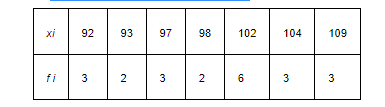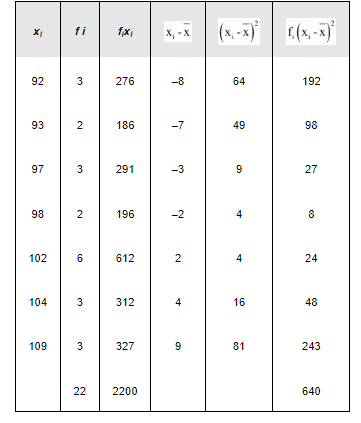Deepak Scored 45->99%ile with Bounce Back Crack Course. You can do it too!

# Find the mean and variance for the data

Question:

Find the mean and variance for the dataSolution:

The data is obtained in tabular form as follows.Here, $N=22, \sum_{i=1}^{7} f_{i} x_{i}=2200$

$\therefore \bar{x}=\frac{1}{N} \sum_{i=1}^{7} f_{i} x_{i}=\frac{1}{22} \times 2200=100$

Variance $\left(\sigma^{2}\right)=\frac{1}{N} \sum_{i=1}^{7} \mathrm{f}_{i}\left(\mathrm{x}_{i}-\overline{\mathrm{x}}\right)^{2}=\frac{1}{22} \times 640=29.09$